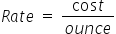Mathematics
Easy

Question

# A perfume costs \$48 for 12-ounce bottle. ____\$ would be the cost of a 2-ounce    bottle.

## 8357Hint:

## The correct answer is: 8

### Cost of 12 ounce perfume bottle is \$48We have to find the rate of per ounce. We will find it by taking the ratio of the cost of cans and number of cans. As the rate is constant, we will use this value to find cost of 2-ounce bottle.So the equation becomeSo the rate = \$4 per ounceLet’s find the cost of 2-ounce bottleWe will rearrange this for cost.For this we will multiply both sides by ounce.Rate × ounce = costCost = Rate × ounceSubstituting the values we have foundCost = 4 × 2Cost = 8Therefore, cost of 2-ounce bottle is \$8

We have to be careful about the units. We have to make sure same quantities have same value.

### Related Questions to study#### With Turito Foundation.#### Get an Expert Advice From Turito.